Question

# 5.) Enter your answer in the provided box. Calculate the following quantity: volume of 1.551 M...

Calculate the following quantity: volume of 1.551 M copper(II) nitrate that must be diluted with water to prepare 946.4 mL of a 0.9513 M solution.

__?___ mL

How many milliliters of 0.836 M HCl are needed to react with 28.8 g of CaCO3?

2HCl(aq) + CaCO3(s) → CaCl2(aq) + CO2(g) + H2O(l)

__?__ mL

 7.) . Enter your answer in the provided box. Sodium hydroxide is used extensively in acid-base titrations because it is a strong, inexpensive base. A sodium hydroxide solution was standardized by titrating 25.47 mL of 0.1070 M standard hydrochloric acid. The initial buret reading of the sodium hydroxide was 1.74 mL, and the final reading was 32.17 mL. What was the molarity of the base solution? __?___M

5)

use dilution formula

M1*V1 = M2*V2

1---> is for stock solution

2---> is for diluted solution

Given:

M1 = 1.551 M

M2 = 0.9513 M

V2 = 946.4 mL

use:

M1*V1 = M2*V2

V1 = (M2 * V2) / M1

V1 = (0.9513*946.4)/1.551

V1 = 580.5 mL

Only 1 question at a time please

#### Earn Coins

Coins can be redeemed for fabulous gifts.

Similar Homework Help Questions
• ### Sodium hydroxide is used extensively in acid-base titrations because it is a strong, inexpensive base. A...

Sodium hydroxide is used extensively in acid-base titrations because it is a strong, inexpensive base. A sodium hydroxide solution was standardized by titrating 36.78 mL of 0.1143 M standard hydrochloric acid. The initial buret reading of the sodium hydroxide was 1.52 mL, and the final reading was 39.50 mL. What was the molarity of the base solution?

• ### Enter your answer in the provided box. Calculate the volume in milliliters of 1.69 M potassium...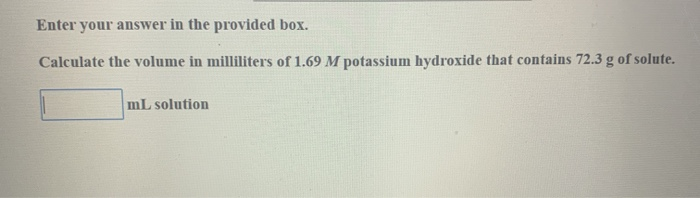Enter your answer in the provided box. Calculate the volume in milliliters of 1.69 M potassium hydroxide that contains 72.3 g of solute. mL solution

• ### Enter your answer in the provided box. Calculate the following quantity: volume of 2.035 M copper(II)...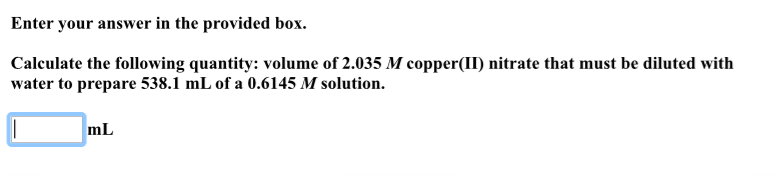Enter your answer in the provided box. Calculate the following quantity: volume of 2.035 M copper(II) nitrate that must be diluted with water to prepare 538.1 mL of a 0.6145 M solution. mL

• ### Enter your answer in the provided box. Calculate the molarity of a solution prepared by diluting...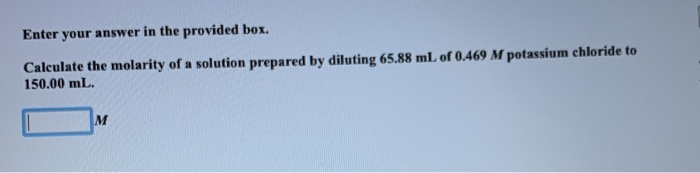Enter your answer in the provided box. Calculate the molarity of a solution prepared by diluting 65.88 mL of 0.469 M potassium chloride to 150.00 mL. IM Enter your answer in the provided box. Calculate the molarity of sodium ion in a solution made by mixing 6.40 mL of 0.619 M sodium chloride with 550.00 mL of 9.02 x 10-2 M sodium sulfate. Assume volumes are additive. M solution

• ### Enter your answer in the provided box. Calculate the composition of the following solution in mass/volume...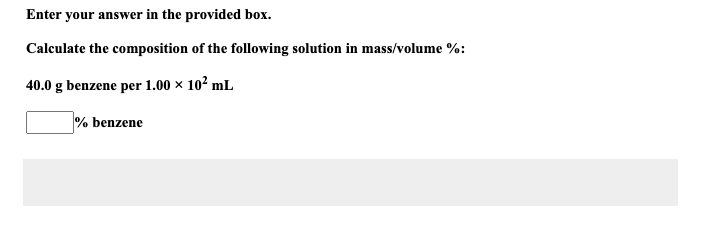Enter your answer in the provided box. Calculate the composition of the following solution in mass/volume %: 40.0 g benzene per 1.00 x 102 mL % benzene Calculate the volume, in L, of water that must be added to dilute 22.9 mL of 10.5 M HCl to 0.0900 M HCl. Enter your answer in the provided box. L water

• ### Enter your answer in the provided box. Calculate the volume of 11.7 M sulfuric acid that...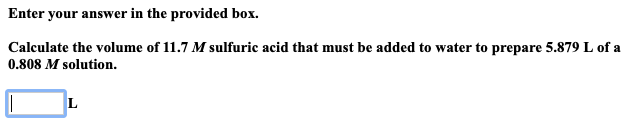Enter your answer in the provided box. Calculate the volume of 11.7 M sulfuric acid that must be added to water to prepare 5.879 L of a 0.808 M solution.

• ### Enter your answer in the provided box. Determine the pH of a 0.16 M solution of...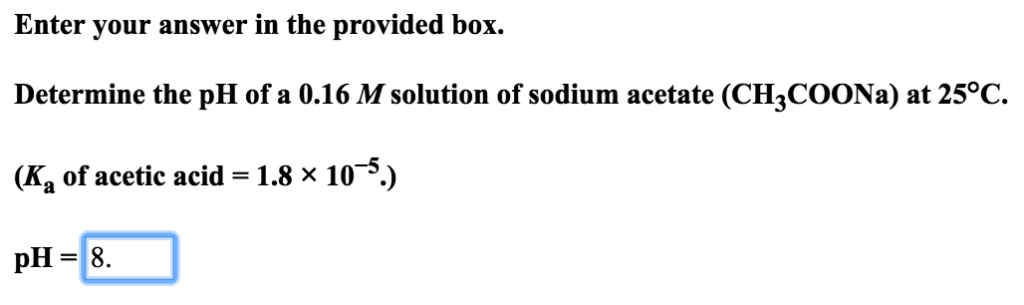Enter your answer in the provided box. Determine the pH of a 0.16 M solution of sodium acetate (CH3COONa) at 25°C. (K, of acetic acid = 1.8 x 10-5.) pH = 8.

• ### Enter your answer in the provided box. If 29.14 mL ofa standard 0.4401 M KOH solution...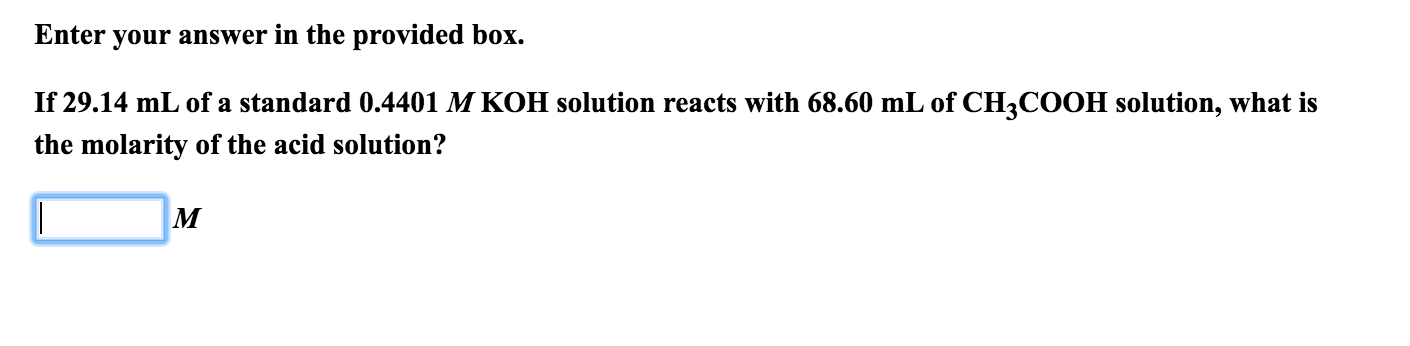Enter your answer in the provided box. If 29.14 mL ofa standard 0.4401 M KOH solution reacts with 68.60 mL of CH3COOH solution, what is the molarity of the acid solution?

• ### Enter your answer in the provided box. A quantity of 2.00 x 102 mL of 0.807...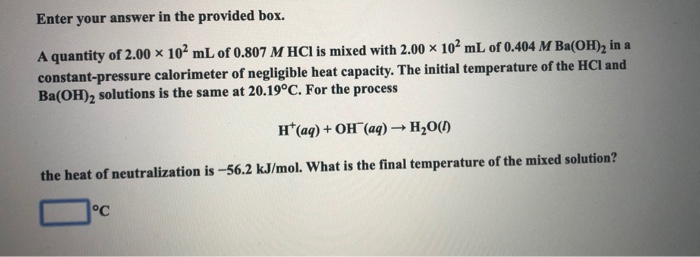Enter your answer in the provided box. A quantity of 2.00 x 102 mL of 0.807 M HCl is mixed with 2.00 10mL of 0.404 M Ba(OH), in a constant-pressure calorimeter of negligible heat capacity. The initial temperature of the HCl and Ba(OH)2 solutions is the same at 20.19°C. For the process H*(aq) + OH(aq) + H2O(1) the heat of neutralization is --56.2 kJ/mol. What is the final temperature of the mixed solution?

• ### Enter your answer in the box provided. Calculate the pH of a 0.65 M sodium formate...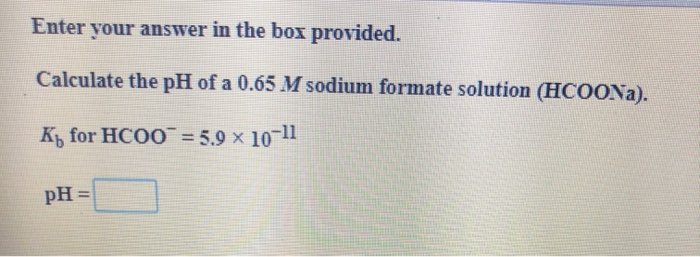Enter your answer in the box provided. Calculate the pH of a 0.65 M sodium formate solution (HCOONa). Ky for HCOO = 5.9 x 10-11 pH =